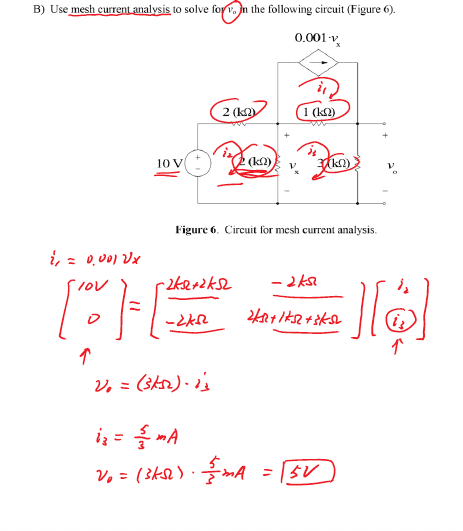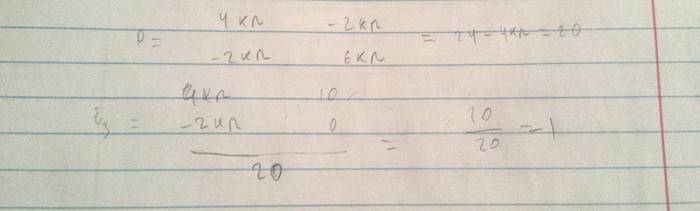# Mesh current equation in matrix form

• vizakenjack

#### vizakenjack

Member warned to use the Formatting Template for homeworki3 -?

This is how I tried to solve using cramer's rule (denominator is the determinant of the matrix, while numerator is the determinant of the modified matrix)I ignored the prefix Kilo, but the method is right. So I get a different value for i3.

Does anyone know what's wrong?

Your mesh equations do not appear to take into account the effects of the controlled current source in loop 1.

Your mesh equations do not appear to take into account the effects of the controlled current source in loop 1.
But it does? You don't need to set up equation for it, because i1 = 0.001Vx

But it does? You don't need to set up equation for it, because i1 = 0.001Vx
So where are it's effects on the rest of the circuit? Hypothetically, suppose it was changed to i1 = 0.003Vx. Shouldn't that change be reflected in your mesh equations? Where would the changes appear?

The controlled current source as a mesh current must create a potential difference across the 1 kΩ resistor in loop 3. You can't just ignore that.

Big hint: To address this, insert an appropriate controlled voltage source in series with the 1 kΩ resistor.

Big hint: To address this, insert an appropriate controlled voltage source in series with the 1 kΩ resistor.

Why would I insert something just randomly? I don't get these riddles!

Why would I insert something just randomly? I don't get these riddles!
It's not random. The controlled voltage source expresses the controlled current source's effect on loop 3. Loop 1's mesh current flows through the 1k resistor of loop 3 and creates a potential drop across it. The inserted voltage source represents that potential drop.